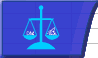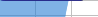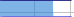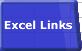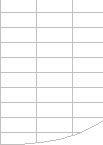# Removing Repeated CalculationsImportance of Speed Faster Calculations Microsoft's Advice Bottlenecks/Size Lookups Dynamic Ranges Totalling Generating Workbooks Multi-Level Calculations Array Formulae SUMIF Example Worksheet Links## Multi-Level and Repeated Calculations:

 It's not the SIZE of your workbook that consumes calculation time, it’s the interpretation, calculation and formatting. Sometimes it's better to have more rows and columns and less complex calculations. It’s also often easier to understand. Many workbooks are built using large formulae containing repeated calculations. Try using additional rows and columns to store calculated intermediate results ONCE and re-use them in other formulae. For example you may have a time-expensive formula where you want the result of an error to be shown as zero. You can write this as: Either A single formula, which is slow: B1=IF(ISERROR(time_expensive_formula),0, time_expensive_formula) Or Two formulae, which is fast: A1=time expensive formula B1=IF(ISERROR(A1),0,A1) Large workbooks are frequently dealing with information at different levels (areas within countries within continents, or products within area within region…). In these cases try to move calculations from the most detailed level to a higher level.© 2001-2020 Decision Models Legal Disclaimer Privacy Statement